集成学习系列目录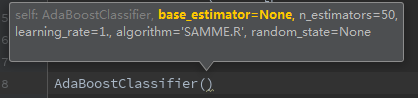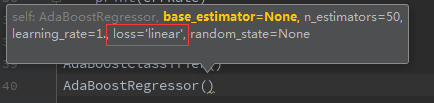1、框架参数

n_estimators: 弱学习器数量，两者都有，一般来说n_estimators太小，容易欠拟合，n_estimators太大，又容易过拟合，一般选择一个适中的数值。默认是50。在实际调参的过程中，常常将n_estimators和下面介绍的参数learning_rate一起考虑。

learning_rate弱学习器的权重缩减系数，取值范围为0~1。对于同样的训练集拟合效果，较小的ν意味着我们需要更多的弱学习器的迭代次数。通常我们用步长和迭代最大次数一起来决定算法的拟合效果。所以这两个参数n_estimators和learning_rate要一起调参。一般来说，可以从一个小一点的ν开始调参，默认是1。

2、弱分类器参数

弱分类器参数要根据所选弱分类器而定，一般使用CART决策树，参数如下：
criterion: 特征选取方法，分类是gini（基尼系数），entropy（信息增益），通常选择gini，即CART算法，如果选择后者，则是ID3和C4,.5；回归是mse或mae，前者是均方差，后者是和均值的差的绝对值之和，一般用前者，因为前者通常更为精准，且方便计算

splitter: 特征划分点选择方法，可以是best或random，前者是在特征的全部划分点中找到最优的划分点，后者是在随机选择的部分划分点找到局部最优的划分点，一般在样本量不大的时候，选择best，样本量过大，可以用random

max_depth: 树的最大深度，默认可以不输入，那么不会限制子树的深度，一般在样本少特征也少的情况下，可以不做限制，但是样本过多或者特征过多的情况下，可以设定一个上限，一般取10~100

min_samples_split:节点再划分所需最少样本数，如果节点上的样本树已经低于这个值，则不会再寻找最优的划分点进行划分，且以结点作为叶子节点，默认是2，如果样本过多的情况下，可以设定一个阈值，具体可根据业务需求和数据量来定

min_samples_leaf: 叶子节点所需最少样本数，如果达不到这个阈值，则同一父节点的所有叶子节点均被剪枝，这是一个防止过拟合的参数，可以输入一个具体的值，或小于1的数（会根据样本量计算百分比）

min_weight_fraction_leaf: 叶子节点所有样本权重和，如果低于阈值，则会和兄弟节点一起被剪枝，默认是0，就是不考虑权重问题。这个一般在样本类别偏差较大或有较多缺失值的情况下会考虑

max_features: 划分考虑最大特征数，不输入则默认全部特征，可以选 log2N,sqrt(N),auto或者是小于1的浮点数（百分比）或整数（具体数量的特征）。如果特征特别多时如大于50，可以考虑选择auto来控制决策树的生成时间

max_leaf_nodes:最大叶子节点数，防止过拟合，默认不限制，如果设定了阈值，那么会在阈值范围内得到最优的决策树，样本量过多时可以设定

min_impurity_decrease/min_impurity_split: 划分最需最小不纯度，前者是特征选择时低于就不考虑这个特征，后者是如果选取的最优特征划分后达不到这个阈值，则不再划分，节点变成叶子节点

以sklearn库自带的手写数据集来分类

from sklearn.model_selection import train_test_split
from sklearn.tree import DecisionTreeClassifier
from sklearn.metrics import confusion_matrix

# ① 分类
data = digits.data
target = digits.target
print(data.shape,target.shape)
x_train,x_test,y_train,y_test = train_test_split(data,target,test_size=0.1)

# 单棵决策树
clf = DecisionTreeClassifier(max_depth=5)
clf.fit(x_train,y_train)
y_pred = clf.predict(x_test)
print("单棵决策树分类结果如下：")
print("混淆矩阵:")
print(confusion_matrix(y_pred,y_test))
print("训练集分数：",clf.score(x_train,y_train))
print("验证集分数：",clf.score(x_test,y_test))

clf.fit(x_train,y_train)
y_pred = clf.predict(x_test)
print("混淆矩阵:")
print(confusion_matrix(y_pred,y_test))
print("训练集分数：",clf.score(x_train,y_train))
print("验证集分数：",clf.score(x_test,y_test))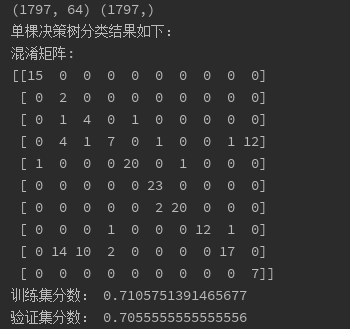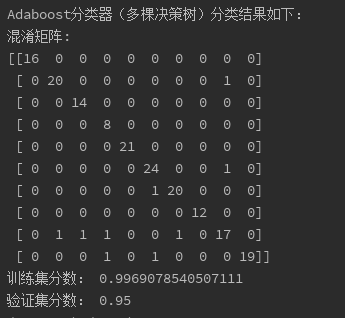以sklearn库自带的波士顿房价数据集来预测

#② 回归
from sklearn.tree import DecisionTreeRegressor
from sklearn import metrics
from sklearn.model_selection import train_test_split

print(data.shape,target.shape)
x_train,x_test,y_train,y_test = train_test_split(data,target,test_size=0.1)

# 单棵回归树
clf = DecisionTreeRegressor(max_depth=5)
clf.fit(x_train,y_train)
y_pred = clf.predict(x_test)
print("单棵回归树结果如下：")
print("训练集分数：",clf.score(x_train,y_train))
print("验证集分数：",clf.score(x_test,y_test))
print("均方误差：",metrics.mean_squared_error(y_test,y_pred))

clf.fit(x_train,y_train)
y_pred = clf.predict(x_test)
print("训练集分数：",clf.score(x_train,y_train))
print("验证集分数：",clf.score(x_test,y_test))
print("均方误差：",metrics.mean_squared_error(y_test,y_pred))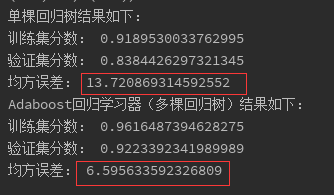展开全文adaboost参数 Adaboost库 sklearnAdaboost算法

3) loss

这个参数只有AdaBoostRegressor有，Adaboost.R2算法需要用到。有线性‘linear’, 平方‘square’和指数 ‘exponential’三种选择，默认是线性，一般使用线性就足够了，除非怀疑这个参数导致拟合程度不好。这个值对应了对第k个弱分类器的中第i个样本的误差的处理，即：如果是线性误差，则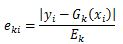；如果是平方误差，则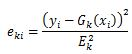；如果是指数误差，则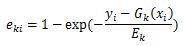，Ek为训练集上的最大误差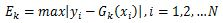5) learning_rate

AdaBoostClassifier和AdaBoostRegressor都有，即每个弱学习器的权重缩减系数ν。加上正则化项后，强学习器的迭代公式为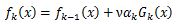。ν的取值范围为0<ν≤1。对于同样的训练集拟合效果，较小的ν意味着需要更多的弱学习器的迭代次数。通常用步长和迭代最大次数一起来决定算法的拟合效果，所以这两个参数n_estimators和learning_rate要一起调参。一般来说，可以从一个小一点的ν开始调参，默认是1。

DecisionTreeClassifier和DecisionTreeRegressor的参数基本类似，这里只拿出调参数时需要尤其注意的最重要几个的参数再拿出来说一遍：

1) 划分时考虑的最大特征数max_features

可以使用很多种类型的值，默认是"None"，意味着划分时考虑所有的特征数；如果是"log2"意味着划分时最多考虑log2(N)个特征；如果是"sqrt"或者"auto"意味着划分时最多考虑sqrt(N)个特征。如果是整数，代表考虑的特征绝对数；如果是浮点数，代表考虑特征百分比，即考虑（百分比xN）取整后的特征数，其中N为样本总特征数。一般来说，如果样本特征数不多，比如小于50，用默认的"None"就可以了；如果特征数非常多，可以灵活使用刚才描述的其他取值来控制划分时考虑的最大特征数，以控制决策树的生成时间。

2) 决策树最大深max_depth

默认可以不输入。如果不输入的话，决策树在建立子树的时候不会限制子树的深度。一般来说，数据少或者特征少的时候可以不管这个值。如果模型样本量多，特征也多的情况下，推荐限制这个最大深度，具体的取值取决于数据的分布。常用的可以取值10-100之间。

3) 内部节点再划分所需最小样本数min_samples_split

这个值限制了子树继续划分的条件，如果某节点的样本数少于min_samples_split，则不会继续再尝试选择最优特征来进行划分。 默认是2，如果样本量不大，不需要管这个值。如果样本量数量级非常大，则推荐增大这个值。

4) 叶子节点最少样本数min_samples_leaf

这个值限制了叶子节点最少的样本数，如果某叶子节点数目小于样本数，则会和兄弟节点一起被剪枝。 默认是1，可以输入最少的样本数的整数，或者最少样本数占样本总数的百分比。如果样本量不大，不需要管这个值；如果样本量数量级非常大，则推荐增大这个值。

5) 叶子节点最小的样本权重和min_weight_fraction_leaf

这个值限制了叶子节点所有样本权重和的最小值，如果小于这个值，则会和兄弟节点一起被剪枝。 默认是0，就是不考虑权重问题。一般来说，如果有较多样本有缺失值，或者分类树样本的分布类别偏差很大，就会引入样本权重，这时就要注意这个值了。

6) 最大叶子节点数max_leaf_nodes

通过限制最大叶子节点数，可以防止过拟合，默认是"None"，即不限制最大的叶子节点数。如果加了限制，算法会建立在最大叶子节点数内最优的决策树。如果特征不多，可以不考虑这个值，但是如果特征分成多的话，可以加以限制，具体的值可以通过交叉验证得到。

首先载入需要的类库：

import numpy as np
import matplotlib.pyplot as plt
%matplotlib inline
from sklearn.tree import DecisionTreeClassifier
from sklearn.datasets import make_gaussian_quantiles
接着生成一些随机数据来做二元分类：

# 生成2维正态分布，生成的数据按分位数分为两类，500个样本,2个样本特征，协方差系数为2
X1, y1 = make_gaussian_quantiles(cov=2.0,n_samples=500, n_features=2,n_classes=2, random_state=1)
# 生成2维正态分布，生成的数据按分位数分为两类，400个样本,2个样本特征均值都为3，协方差系数为2
X2, y2 = make_gaussian_quantiles(mean=(3, 3), cov=1.5,n_samples=400, n_features=2, n_classes=2, random_state=1)
# 将两组数据合成一组数据
X = np.concatenate((X1, X2))
y = np.concatenate((y1, - y2 + 1))
通过可视化看看分类数据，它有两个特征，两个输出类别，用颜色区别。
plt.scatter(X[:, 0], X[:, 1], marker='o', c=y)
输出为下图：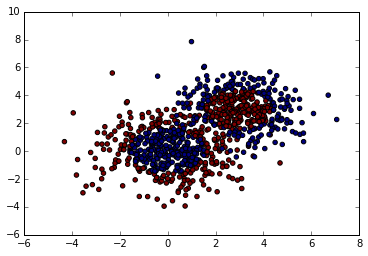bdt = AdaBoostClassifier(DecisionTreeClassifier(max_depth=2, min_samples_split=20, min_samples_leaf=5), algorithm="SAMME",n_estimators=200, learning_rate=0.8)
bdt.fit(X, y)
这里选择了SAMME算法，最多200个弱分类器，步长0.8，在实际运用中可能需要通过交叉验证调参而选择最好的参数。拟合完了后，用网格图来看看它拟合的区域。
x_min, x_max = X[:, 0].min() - 1, X[:, 0].max() + 1
y_min, y_max = X[:, 1].min() - 1, X[:, 1].max() + 1
xx, yy = np.meshgrid(np.arange(x_min, x_max, 0.02),
np.arange(y_min, y_max, 0.02))

Z = bdt.predict(np.c_[xx.ravel(), yy.ravel()])
Z = Z.reshape(xx.shape)
cs = plt.contourf(xx, yy, Z, cmap=plt.cm.Paired)
plt.scatter(X[:, 0], X[:, 1], marker='o', c=y)
plt.show()
输出的图如下：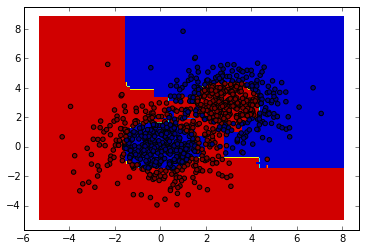print "Score:", bdt.score(X,y)
输出为：
Score: 0.913333333333
也就是说拟合训练集数据的分数还不错。当然分数高并不一定好，因为可能过拟合。

现在将最大弱分离器个数从200增加到300，再来看看拟合分数。

bdt = AdaBoostClassifier(DecisionTreeClassifier(max_depth=2, min_samples_split=20, min_samples_leaf=5), algorithm="SAMME",n_estimators=300, learning_rate=0.8)
bdt.fit(X, y)
print "Score:", bdt.score(X,y)
此时的输出为：
Score: 0.962222222222
这印证了前面讲的，弱分离器个数越多，则拟合程度越好，当然也越容易过拟合。

现在降低步长，将步长从上面的0.8减少到0.5，再来看看拟合分数。

bdt = AdaBoostClassifier(DecisionTreeClassifier(max_depth=2, min_samples_split=20, min_samples_leaf=5),algorithm="SAMME", n_estimators=300, learning_rate=0.5)
bdt.fit(X, y)
print "Score:", bdt.score(X,y)
此时的输出为：
Score: 0.894444444444
可见在同样的弱分类器的个数情况下，如果减少步长，拟合效果会下降。

最后看看当弱分类器个数为700，步长为0.7时候的情况：

bdt = AdaBoostClassifier(DecisionTreeClassifier(max_depth=2, min_samples_split=20, min_samples_leaf=5),algorithm="SAMME",n_estimators=600, learning_rate=0.7)
bdt.fit(X, y)
print "Score:", bdt.score(X,y)
此时的输出为：
Score: 0.961111111111
此时的拟合分数和最初的300弱分类器，0.8步长的拟合程度相当。也就是说，在这个例子中，如果步长从0.8降到0.7，则弱分类器个数要从300增加到700才能达到类似的拟合效果。

展开全文机器学习 AdaBoost

千次阅读 2015-03-12 11:23:07虽然弱分类器的样式没有限制，可以是基于多维特征的决策树，甚至是SVM，但通常每个弱分类器都是基于所有特征中的某一维构建，并且输出的结果只有+1，-1两种（对于二分类）。因此，在训练时，每一轮迭代都挑选在当前训练集分布下，分类效果最好的那一维特征对应的弱分类器。三、举例说明

插曲：：

文献中记录的.M1算法
初始
1.获得一组样本(X)和它的分类(Y)和一个分类器(weaklearn).
2.赋予平均的权值分布D(i)
进入循环:T次
1. 赋予弱分类器权值D(i),使用弱分类器获得样本(X)到分类(Y)上的一个映射.(就是把某个X归到某个Y类中去)
2. 计算这个映射的误差e.e=各个归类错误的样本权值之和.如果e>1/2那么弱分类器训练失败,挑出循环,训练结束(这在二值检测中是不会发生的,而多值的情况就要看分类器够不够强健了)
3. 设B = e / ( 1 - e ).用于调整权值.因为e<1/2.因此0<B<1
4. 如果某样本分类正确,该样本的权值就乘以B让权值变小;如果分类错误,就让该样本的权值乘以B^-1或者不变,这样就让分类正确的样本权值降低,分类错误的样本权值升高,加强了对较难分类样本的分类能力
5. 权值均衡化
循环结束
1. 最终的分类器是,当一个X进入时,遍历所有Y,寻找使(h(x)=y的情况下,log(1/B)之和)最大者即是输出分类y

展开全文集成方法（ensemble method）

要获得好的集成，个体学习器应“好而不同”，即个体学习器要有一定的准确性（不能太坏），并且要有多样性（学习器间具有差异）。

集成方法主要可分为
个体学习器存在强依赖关系，必须串行生成的序列化方法（Boosting），
个体学习器不存在强依赖关系，可同时并行化方法（Bagging）。

• bagging 基于数据重抽样的分类器构建方法

在Bagging方法中，主要通过对训练数据集进行随机采样，以重新组合成不同的数据集，新数据集和旧数据集大小相等，利用弱学习算法对不同的新数据集进行学习，得到一系列的预测结果，对这些预测结果做平均或者投票做出最终的预测。注：随机森林算法是基于Bagging思想的机器学习算法。

• boosting

boosting和bagging很类似，但是boosting的分裂结果是基于所有分类器的加权求和结果的，每个权重代表的是对应分类器在上一轮迭代的成功度，而bagging的权重是相等的。

这族的算法工作机制蕾丝：先从初始训练集训练出一个集学习器。再根据基学习器的表现对样本分布进行调整，是的先前基学习器做错的训练样本在后续受到更多关注，然后基于调整后的样本分布训练下一个基学习器；如此反复，直到达到某个预定的足够小的错误率或达到预先指定的最大迭代次数T，最后讲这些基学习器进行加权结合。

能否用弱分类器和多个实力来构建一个强分类器，前一个基本分类器分错的样本会得到加强，加权后的全体样本再次被用来训练下一个基本分类器。同时，在每一轮中加入一个新的弱分类器，直到达到某个预定的足够小的错误率或达到预先指定的最大迭代次数。

• 初始化训练数据的权值分布。如果有N个样本，则每一个训练样本最开始时都被赋予相同的权重：1/N。
• 训练弱分类器。具体训练过程中，如果某个样本点已经被准确地分类，那么在构造下一个训练集中，它的权重就被降低；相反，如果某个样本点没有被准确地分类，那么它的权重就得到提高。然后，权重更新过的样本集被用于训练下一个分类器，整个训练过程如此迭代地进行下去。
• 将各个训练得到的弱分类器组合成强分类器。各个弱分类器的训练过程结束后，加大分类误差率小的弱分类器的权重，使其在最终的分类函数中起着较大的决定作用，而降低分类误差率大的弱分类器的权重，使其在最终的分类函数中起着较小的决定作用。换言之，误差率低的弱分类器在最终分类器中占的权重较大，否则较小。

算法流程

步骤1. 首先，初始化训练数据的权值分布。每一个训练样本最开始时都被赋予相同的权重：1/N。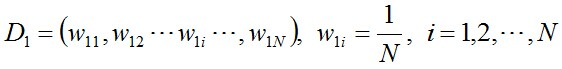步骤2. 进行多轮迭代，用m = 1,2, …, M表示迭代的第多少轮

a.使用具有权值分布Dm的训练数据集学习，得到基本分类器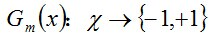b. 计算Gm(x)在训练数据集上的分类误差率即：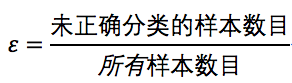由上述式子可知，Gm(x)在训练数据集上的误差率em就是被Gm(x)误分类样本的权值之和。

c. 计算Gm(x)的系数，am表示Gm(x)在最终分类器中的重要程度（目的：得到基本分类器在最终分类器中所占的权重）：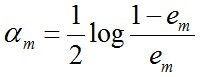由上述式子可知，em <= 1/2时，am >= 0，且am随着em的减小而增大，意味着分类误差率越小的基本分类器在最终分类器中的作用越大。

d. 更新训练数据集的权值分布（目的：得到样本的新的权值分布），用于下一轮迭代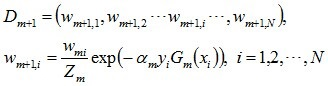其中，Zm是规范化因子，使得Dm+1成为一个概率分布：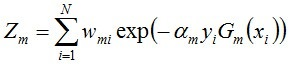步骤3. 组合各个弱分类器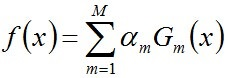从而得到最终分类器，如下：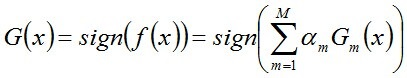原理和例子

基于单层决策树构建弱分类器

单层决策树（decision stump）是一种简单的决策树桩

准备数据集

from numpy import *
from operator import*
import matplotlib.pyplot as plt

datMat = matrix([[ 1. ,  2.1],
[ 2. ,  1.1],
[ 1.3,  1. ],
[ 1. ,  1. ],
[ 2. ,  1. ]])
classLabels = [1.0, 1.0, -1.0, -1.0, 1.0]
return datMat,classLabels

画出来数据集的示意图：

def showScatter(matrix, labels):
plt.figure(figsize=(8,6))
x1 = []; y1 = []; x2 = []; y2 = []
for i in range(len(labels)):
if labels[i] == 1.0:
x1.append(matrix[i, 0])
y1.append(matrix[i, 1])
else:
x2.append(matrix[i, 0])
y2.append(matrix[i, 1])
plt.scatter(x1, y1, marker='o',color='g', alpha=0.7, label='1.0')
plt.scatter(x2, y2, marker='^',color='red', alpha=0.7, label='-1.0')
plt.title('dataset')
plt.ylabel('variable Y')
plt.xlabel('Variable X')
plt.legend(loc='upper right')
plt.show()

showScatter(datmat,classlabels)

如图所示，我们要把两种图形分开：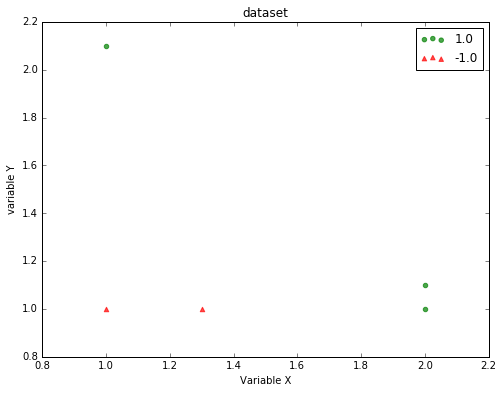我们将多个单层决策树，构建一个对该数据集完全正确分类的分类器。

单层决策树生成函数

伪代码：

• 将最小错误率minError设为Inf(正无穷)
• 对于数据集的每一个特征：（第一层）
• 对每个补步长：（第二层）
• 对每个不等号：（第三层）
建立一颗决策树并用加权数据集对它进行测试
如果错误率低于minError,将当前决策树设为最佳单层决策树
• 返回最佳单层决策树

（共三层循环）

'''
通过阈值比较对数据进行分类的，所有在阈值一边的数据分到类别-1，而在另一边的数据分到类别1
'''
# dataMatirx：要分类的数据
# dimen：维度
# threshVal：阈值
# threshIneq：有两种，‘lt’=lower than，‘gt’=greater than
def stumpClassify(dataMatrix,dimen,threshVal,threshIneq):#just classify the data
retArray = ones((shape(dataMatrix),1))
if threshIneq == 'lt':
retArray[dataMatrix[:,dimen] <= threshVal] = -1.0
else:
retArray[dataMatrix[:,dimen] > threshVal] = -1.0
return retArray
#beststump存储给定权重向量D时所得到的的最佳单层决策树的相关信息
#numSteps 用于在特征的所有可能值上进行便利
#bestClasEst 最佳单层决策树的判断
def buildStump(dataArr,classLabels,D):
dataMatrix = mat(dataArr); labelMat = mat(classLabels).T
m,n = shape(dataMatrix)
numSteps = 10.0; bestStump = {}; bestClasEst = mat(zeros((m,1)))
minError = inf #init error sum, to +infinity
for i in range(n):#loop over all dimensions
rangeMin = dataMatrix[:,i].min(); rangeMax = dataMatrix[:,i].max();
stepSize = (rangeMax-rangeMin)/numSteps
for j in range(-1,int(numSteps)+1):#loop over all range in current dimension
for inequal in ['lt', 'gt']: #go over less than and greater than
threshVal = (rangeMin + float(j) * stepSize)
predictedVals = stumpClassify(dataMatrix,i,threshVal,inequal)#call stump classify with i, j, lessThan
errArr = mat(ones((m,1)))
errArr[predictedVals == labelMat] = 0
weightedError = D.T*errArr  #calc total error multiplied by D
#print ("split: dim %d, thresh %.2f, thresh ineqal: %s, the weighted error is %.3f" % (i, threshVal, inequal, weightedError))
if weightedError < minError:
minError = weightedError
bestClasEst = predictedVals.copy()
bestStump['dim'] = i
bestStump['thresh'] = threshVal
bestStump['ineq'] = inequal
return bestStump,minError,bestClasEst

拟运行一下看看~

D=mat(ones((5,1))/5)
buildStump(datmat,classlabels,D)
({'dim': 0, 'ineq': 'lt', 'thresh': 1.3}, matrix([[ 0.2]]), array([[-1.],
[ 1.],
[-1.],
[-1.],
[ 1.]]))

伪代码：

• 对每次迭代：
利用buildStump()函数找到最佳的单层决策树
将最佳单层决策树加入到单层决策树组
计算alpha
计算新的权重向量D
更新累计类别估计值
如果错误率大于0.0，退出循环
#函数尾部DS代表使用单层决策树为弱分类器
#aggClassEst记录每个数据点的类别估计累加值
weakClassArr = []
m = shape(dataArr)
D = mat(ones((m,1))/m)   #init D to all equal
aggClassEst = mat(zeros((m,1)))
for i in range(numIt):
bestStump,error,classEst = buildStump(dataArr,classLabels,D)#build Stump
#print("D:",D.T)
alpha = float(0.5*log((1.0-error)/max(error,1e-16)))#calc alpha, throw in max(error,eps) to account for error=0
bestStump['alpha'] = alpha
weakClassArr.append(bestStump)                  #store Stump Params in Array
#print ("classEst: ",classEst.T)
expon = multiply(-1*alpha*mat(classLabels).T,classEst) #exponent for D calc, getting messy
D = multiply(D,exp(expon))                              #Calc New D for next iteration
D = D/D.sum()
#calc training error of all classifiers, if this is 0 quit for loop early (use break)
aggClassEst += alpha*classEst
#print ("aggClassEst: ",aggClassEst.T)
aggErrors = multiply(sign(aggClassEst) != mat(classLabels).T,ones((m,1)))
errorRate = aggErrors.sum()/m
# print ("total error: ",errorRate)
if errorRate == 0.0: break
return weakClassArr,aggClassEst

其中sign函数 -1 if x < 0, 0 if x==0, 1 if x > 0
multiply(3==4,1) >>>0
multiply(3==3,1) >>>1

测试以上程序：

classifierArray
Out:
([{'alpha': 0.6931471805599453, 'dim': 0, 'ineq': 'lt', 'thresh': 1.3},
{'alpha': 0.9729550745276565, 'dim': 1, 'ineq': 'lt', 'thresh': 1.0},
{'alpha': 0.8958797346140273,
'dim': 0,
'ineq': 'lt',
'thresh': 0.90000000000000002}],
matrix([[ 1.17568763],
[ 2.56198199],
[-0.77022252],
[-0.77022252],
[ 0.61607184]]))

#datToClass待分类的样例
#clssifierArr 弱分类器组成的数组
dataMatrix = mat(datToClass)#do stuff similar to last aggClassEst in adaBoostTrainDS
m = shape(dataMatrix)
aggClassEst = mat(zeros((m,1)))
for i in range(len(classifierArr)):
classEst = stumpClassify(dataMatrix,classifierArr[i]['dim'],\
classifierArr[i]['thresh'],\
classifierArr[i]['ineq'])#call stump classify
aggClassEst += classifierArr[i]['alpha']*classEst
#print (aggClassEst)
return sign(aggClassEst)

看看实际运行结果

matrix([[ 1.],
[-1.],
[ 1.],
[ 1.]])

1. 收集数据
2. 准备数据：确保类别是+1和-1
3. 分析数据
5. 测试算法：对测试集合
6. 使用算法

errArr = mat(ones((67,1)))
errArr[prediction10!=mat(testLabelArr).T].sum()/67
0.23880597014925373
分类器数目训练错误率测试错误率
10.280.27
100.230.24
500.190.21
1000.190.22
5000.160.25
10000.140.31

存在过拟合现象。
回到数据集本身，考虑将缺失的值不化为0而转化为其他值，如平均值等，再次尝试。

常用的组合

BaggingClassifier： Bagging分类器组合
BaggingRegressor： Bagging回归器组合
ExtraTreeClassifier：ExtraTree分类器组合
ExtraTreeRegressor： ExtraTree回归器组合
RandomTreeClassifier：随机森林分类器组合
RandomTreeRegressor： 随机森林回归器组合
使用类：

参数介绍：

- base_estimator :(default=DecisionTreeClassifier)
选择哪种若分类器，scikit-learn中的分类器都可以

- n_estimators :integer, (default=50)
最大迭代次数，一般来说n_estimators太小，容易欠拟合，n_estimators太大，又容易过拟合，一般选择一个适中的数值。默认是50。在实际调参的过程中，我们常常将n_estimators和下面介绍的参数learning_rate一起考虑。

- learning_rate :float, (default=1.0) 迭代次数的每个弱分类器权重设置参数

- algorithm : {‘SAMME’, ‘SAMME.R’},(default=’SAMME.R’)SAMME.R’的话弱分类器需要支持概率预测，比如逻辑回归；‘SAMME’这个是针对离散的变量。

类中方法：
fit(X, y[, sample_weight]) #拟合模型
predict(X)                 #求预测值
predict_proba(X)
score(X, y[, sample_weight])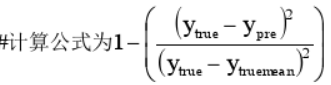参数：

- base_estimator :(default=DecisionTreeClassifier)
选择哪种若分类器，scikit-learn中的分类器都可以

- learning_rate :float, (default=1.0) 迭代次数的每个弱分类器权重设置参数

- n_estimators :integer, (default=50)
最大迭代次数，一般来说n_estimators太小，容易欠拟合，n_estimators太大，又容易过拟合，一般选择一个适中的数值。默认是50。在实际调参的过程中，我们常常将n_estimators和下面介绍的参数learning_rate一起考虑
DecisionTreeClassifier和DecisionTreeRegressor的参数重要的如下：

1) 划分时考虑的最大特征数max_features: 可以使用很多种类型的值，默认是"None",意味着划分时考虑所有的特征数；如果是"log2"意味着划分时最多考虑log2Nlog2N个特征；如果是"sqrt"或者"auto"意味着划分时最多考虑N−−√N个特征。如果是整数，代表考虑的特征绝对数。如果是浮点数，代表考虑特征百分比，即考虑（百分比xN）取整后的特征数。其中N为样本总特征数。一般来说，如果样本特征数不多，比如小于50，我们用默认的"None"就可以了，如果特征数非常多，我们可以灵活使用刚才描述的其他取值来控制划分时考虑的最大特征数，以控制决策树的生成时间。

2) 决策树最大深max_depth: 默认可以不输入，如果不输入的话，决策树在建立子树的时候不会限制子树的深度。一般来说，数据少或者特征少的时候可以不管这个值。如果模型样本量多，特征也多的情况下，推荐限制这个最大深度，具体的取值取决于数据的分布。常用的可以取值10-100之间。

3) 内部节点再划分所需最小样本数min_samples_split: 这个值限制了子树继续划分的条件，如果某节点的样本数少于min_samples_split，则不会继续再尝试选择最优特征来进行划分。 默认是2.如果样本量不大，不需要管这个值。如果样本量数量级非常大，则推荐增大这个值。

4) 叶子节点最少样本数min_samples_leaf: 这个值限制了叶子节点最少的样本数，如果某叶子节点数目小于样本数，则会和兄弟节点一起被剪枝。 默认是1,可以输入最少的样本数的整数，或者最少样本数占样本总数的百分比。如果样本量不大，不需要管这个值。如果样本量数量级非常大，则推荐增大这个值。

5）叶子节点最小的样本权重和min_weight_fraction_leaf：这个值限制了叶子节点所有样本权重和的最小值，如果小于这个值，则会和兄弟节点一起被剪枝。 默认是0，就是不考虑权重问题。一般来说，如果我们有较多样本有缺失值，或者分类树样本的分布类别偏差很大，就会引入样本权重，这时我们就要注意这个值了。

6) 最大叶子节点数max_leaf_nodes: 通过限制最大叶子节点数，可以防止过拟合，默认是''None''，即不限制最大的叶子节点数。如果加了限制，算法会建立在最大叶子节点数内最优的决策树。如果特征不多，可以不考虑这个值，但是如果特征分成多的话，可以加以限制，具体的值可以通过交叉验证得到。
# -*- encoding:utf-8 -*-

'''
本例是Sklearn网站上的关于决策树桩、决策树、
在分类上的错误率。
这个例子基于Sklearn.datasets里面的make_Hastie_10_2数据库。
取了12000个数据，其他前10000个作为训练集，后面2000个作为了测试集。
'''

import numpy as np
import matplotlib.pyplot as plt
from sklearn import datasets
from sklearn.tree import DecisionTreeClassifier
from sklearn.metrics import zero_one_loss
import time
a=time.time()

n_estimators=400
learning_rate=1.0
X,y=datasets.make_hastie_10_2(n_samples=12000,random_state=1)
X_test,y_test=X[10000:],y[10000:]
X_train,y_train=X[:2000],y[:2000]

#决策树桩
dt_stump=DecisionTreeClassifier(max_depth=1,min_samples_leaf=1)
dt_stump.fit(X_train,y_train)
dt_stump_err=1.0-dt_stump.score(X_test,y_test)

#决策树
dt=DecisionTreeClassifier(max_depth=9,min_samples_leaf=1)
dt.fit(X_train,y_train)
dt_err=1.0-dt.score(X_train,y_test)

#决策树桩的生成
n_estimators=n_estimators,algorithm='SAMME')

fig=plt.figure()
ax.plot([1,n_estimators],[dt_stump_err]*2,'k-',label='Decision Stump Error')
ax.plot([1,n_estimators],[dt_err]*2,'k--',label='Decision Tree Error')

ax.set_ylim((0.0,0.5))
ax.set_xlabel('n_estimators')
ax.set_ylabel('error rate')

leg=ax.legend(loc='upper right',fancybox=True)
leg.get_frame().set_alpha(0.7)
b=time.time()
print ('total running time of this example is :',(b-a))
plt.show()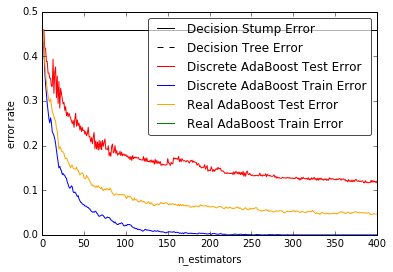展开全文python 算法算法 决策树 python 机器学习 人工智能
• AdaBoost算法流程 输入: 训练集 T={(x1,y1),(x2,y2),…,(xN,yN)} AdaBoost算法在初始时会给每一个样本一个权值 1 初始权值为 1/N 2 M是设定的一个迭代轮次 每迭代一轮都会生成一个弱分类器， 我们需要迭代 第2步–...adaboost算法python 深度学习 算法Adaboost
• viola-jones论文Robust Real-Time Face Detection中的 haar+Adaboost人脸检测方法、人脸检测样本正负样本，人脸2000+，非人脸4000+Adaboost
• 1.2 代码实现 数据集采用的是sklearn提供的鸢尾花数据集，这个数据集用过很多次了，此处就不对该数据集作过多介绍。 from sklearn.datasets import load_iris from sklearn.model_selection import train_test_...python 开发语言 后端Adaboost boosting Adaboost算法机器学习 python 数据

2018-11-04 21:05:40...